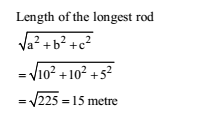# RRB ALP 2018 Practice Test Papers | Arithmetic Questions (Day-42)

Dear Aspirants, Here we have given the Important RRB ALP & Technicians Exam 2018 Practice Test Papers. Candidates those who are preparing for RRB ALP 2018 can practice these Arithmetic Questions to get more confidence to Crack RRB 2018 Examination.

[WpProQuiz 2204]

Click “Start Quiz” to attend these Questions and view Explanation

1. If A and B together can complete a piece of work in 15 days and B alone in 20 days, in how many days can A alone complete the work?

(a) 60

(b) 45

(c) 40

(d) 30

1. By walking at 3/ 4 of his usual speed, a man reaches his office 20 minutes later than his usual time. The usual time taken by him to reach his office is

(a) 75 minutes

(b) 60 minutes

(c) 40 minutes

(d) 30 minutes

1. The length (in metres) of the longest rod that can be put in a room of dimensions 10 m × 10 m × 5 m is

(a) 15√ 3

(b) 15

(c) 10√ 2

(d) 5√3

1. The ninth term of the sequence 0, 3, 8, 15, 24, 35,…. is

(a) 63

(b) 70

(c) 80

(d) 99

1. A number, when divided by 114, leaves remainder 21. If the same number is divided by 19, then the remainder will be

(a) 1

(b) 2

(c) 7

(d) 17

1. Two numbers are in the ratio 3: 4. Their L.C.M. is 84. The greater number is

(a) 21

(b) 24

(c) 28

(d) 84

1. If a and b be positive integers such that a2 – b2 = 19, then the value of a is

(a) 19

(b) 20

(c) 9

(d) 10

1. Two natural numbers are in the ratio 3: 5 and their product is 2160. The smaller of the numbers is

(a) 36

(b) 24

(c) 18

(d) 12

1. The average of odd numbers upto 100 is

(a) 50.5

(b) 50

(c) 49.5

(d) 49

1. 1.14 expressed as a per cent of 1.9 is

(a) 6%

(b) 10%

(c) 60%

(d) 90%

(A + B)’s 1 day’s work= 1/ 15

B’s 1 day’s work =1 /20

A’s 1 day’s work=1 /15-1/ 20 =1/60

A alone will do the work in 60 days

4/ 3 of usual time = Usual time + 20 minutes

1/3 rd of usual time = 20 minutes

Usual time 20x 3 = 60 minutes

1. Answer: (b)0 + 3 = 3

3 + 5 = 8

8 + 7 = 15

15 + 9 = 24

24 + 11 = 35

35 + 13 = 48

48 + 15 = 63

63 + 17 = 80

If the first divisor is a multiple of second divisor.Then, remainder by the second divisor.

Remainder = 21 /19 = 2

Let the numbers be 3x and 4x.

Their LCM = 12x

12x = 84

x =84/12 =7

Larger number = 4x = 4 × 7 = 28

a2 – b2 = 19 => 102 – 92 = 19 => a = 10

Let the numbers be 3x and 5x.

3x × 5x = 2160

X2=2160/(3×5)=144=>x = 12

Smaller number = 3x = 3 × 12 = 36

Average of the first n natural odd numbers = n

Number of odd numbers upto 100 = 50 = required average.

No. of odd no upto 100 = 50

Sum of 50 odd no = 502

Average = 50 x50/ 50  = 50.

Required percentage 1.14/1.9x 100= 60%

RRB ALP 2018 – “All in One” Study Materials and Practice Sets